Programming Fundamentals
Lecture 05 Loops, Arrays and Images
Edirlei Soares de Lima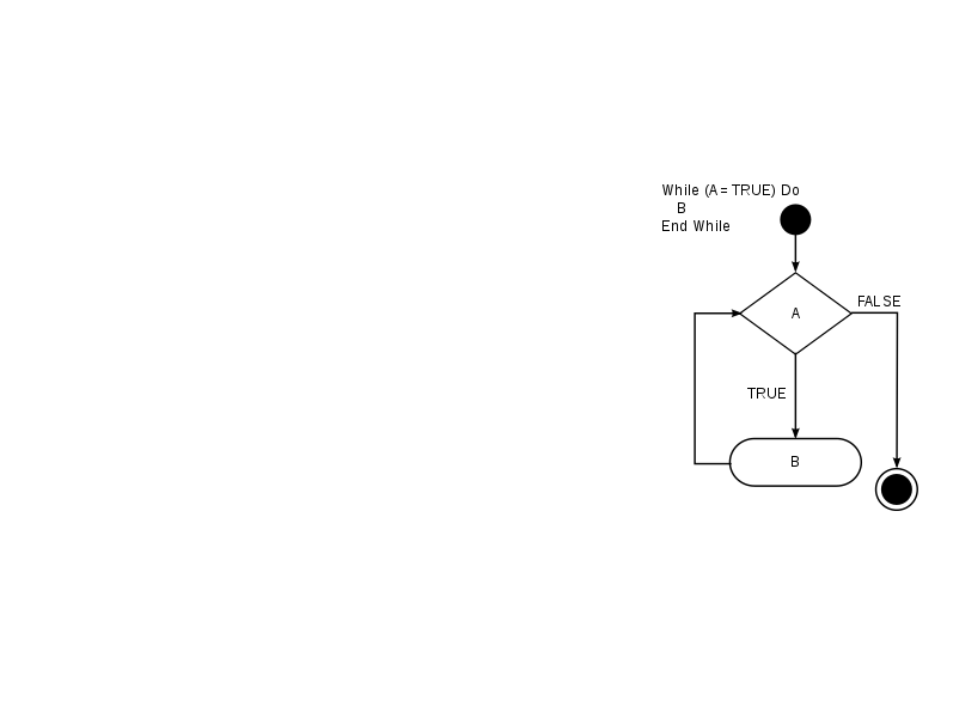Loop Statements
You may encounter situations where a
block of code needs to be executed
several times.
A loop statement allows us to execute a
statement or group of statements
multiple times.
Lua loop statements: while, for, repeatLoop Statements (while)
The while consists of a block of code and a boolean condition.
The condition is evaluated, and if it is true, the code within
the block is executed. This repeats until the condition
becomes false.
While the “boolean condition” is true,
the “block of code” is executed.
.
..
while boolean_condition do
- block of code
-
When the condition becomes false, the
execution of the program continues to
the code that comes after the block of
code of the while.
end
...Loop Statements Example 1
Examplo 1:
local i = 0
write all numbers between 0
and 100 on screen.”
Implement a program to
while i <= 100 do
io.write(i, "\n")
i = i + 1
end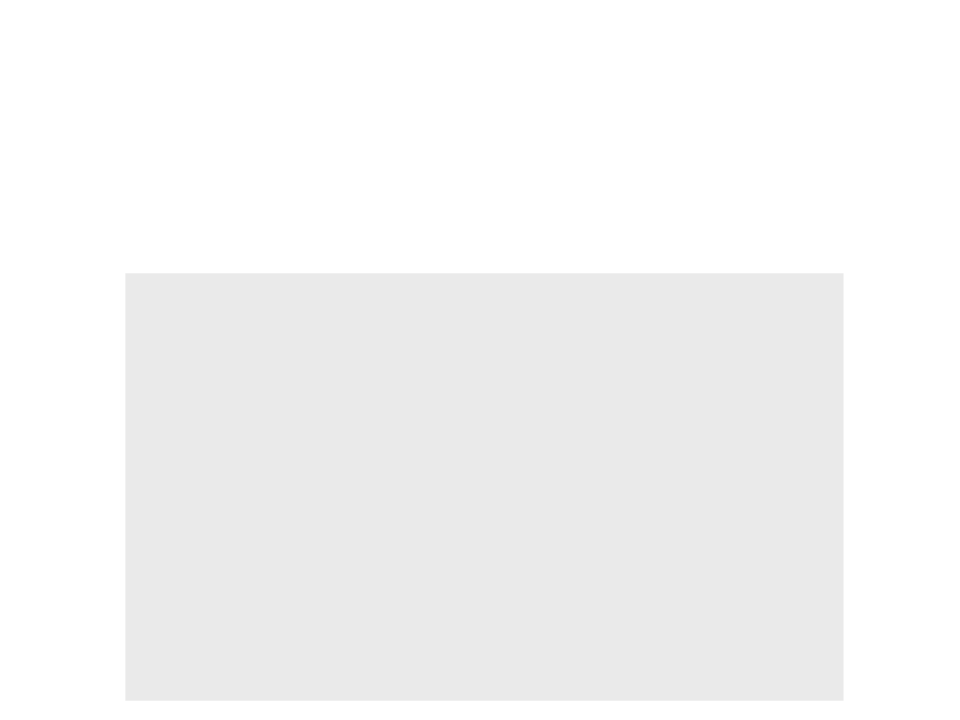Loop Statements Example 2
Example 2: Write a program to read 10 numbers and find their
sum and average”
local i = 0
local sum = 0
local avg = 0
io.write("Input the 10 numbers:\n");
while i < 10 do
sum = sum + value
i = i + 1
end
avg = sum / 10.0
io.write("The sum is: ", sum, "\n")
io.write("The Average is: ", avg, "\n")Loop Statements (for)
Another common loop statement is the for.
It simplifies the implementation of loops that need to be executed a
specific number of times.
...
for var = initial_value, final_value, increment do
- block of code
-
end
...
The block of code will be executed for each value of var,
starting from initial_valueand going to final_value,
using incrementto increment/decrement the value of var.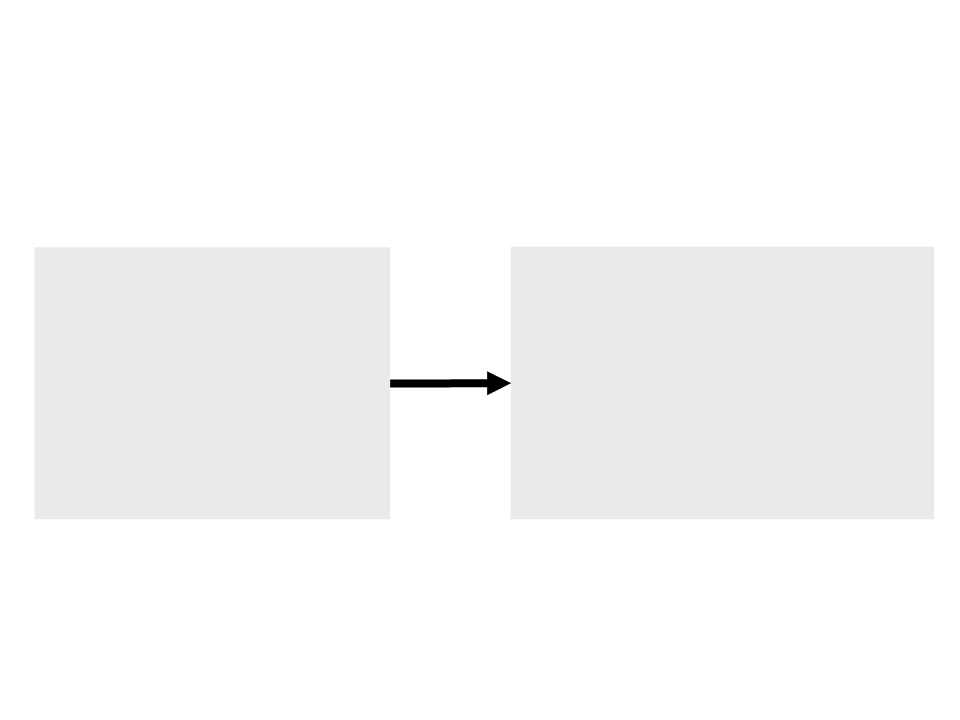Loop Statements Example 1
Example 1: “Write all numbers between 0 and 100 on screen.”
local i = 0
while i <= 100 do
io.write(i, "\n")
i = i + 1
for i = 0, 100, 1 do
io.write(i, "\n")
end
end
Important:
In the for statement, the control variable i is local variable;
Never change the value of control variable manually;Loop Statements Example 2
Example 2: Write a program to read 10 numbers and find their
sum and average”
local sum = 0
local avg = 0
io.write("Input the 10 numbers:\n");
for i = 1, 10, 1 do
sum = sum + value
end
avg = sum / 10.0
io.write("The sum is: ", sum, "\n")
io.write("The Average is: ", avg, "\n")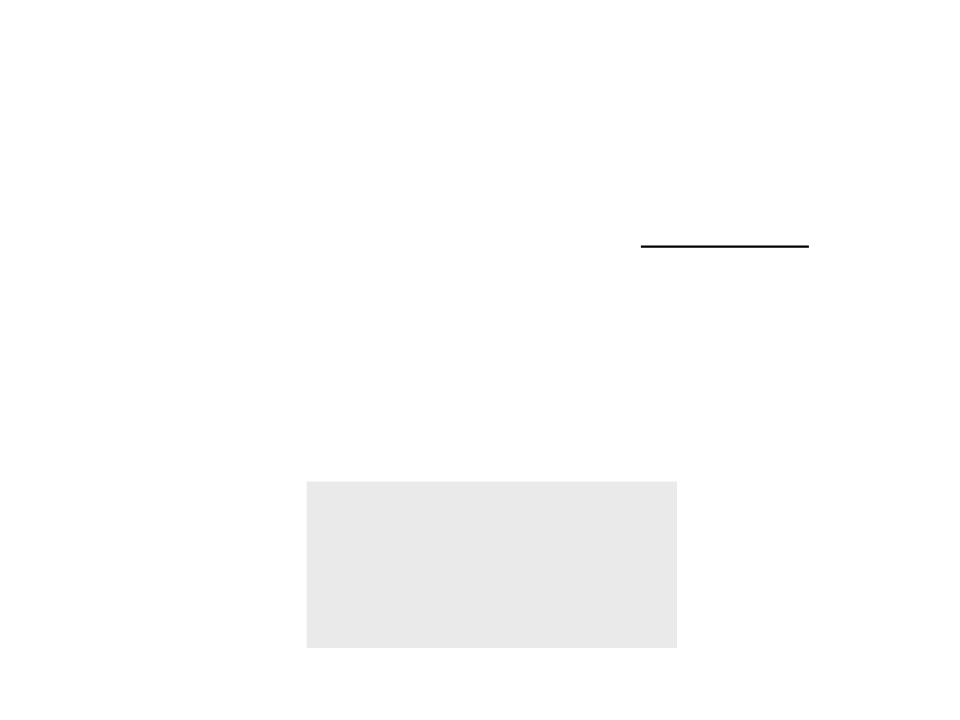Loop Statements (repeat)
The while loop checks the condition before executing the
block of code. Thus, it is often known as a pre-test loop.
Lua offers a third loop statement called repeat:
The condition is evaluated after the execution of block of code.
This means that the block of code will be executed at least once.
...
repeat
-- block of code
until boolean condition
...Loop Statements Example 1
Example 1: Write a program to read 10 numbers and find their
sum and average”
local i = 1
local sum = 0
local avg = 0
io.write("Input the 10 numbers:\n");
repeat
sum = sum + value
i = i + 1
until i > 10
avg = sum / 10.0
io.write("The sum is: ", sum, "\n")
io.write("The Average is: ", avg, "\n")Back to the “Hello World”
In the last version of the “Hello World”, we moved the text and
of the screen.
What if we need to do the same with 20 “Hello World’s” at the
same time?
Duplicating code is never a good option!
How can we do that?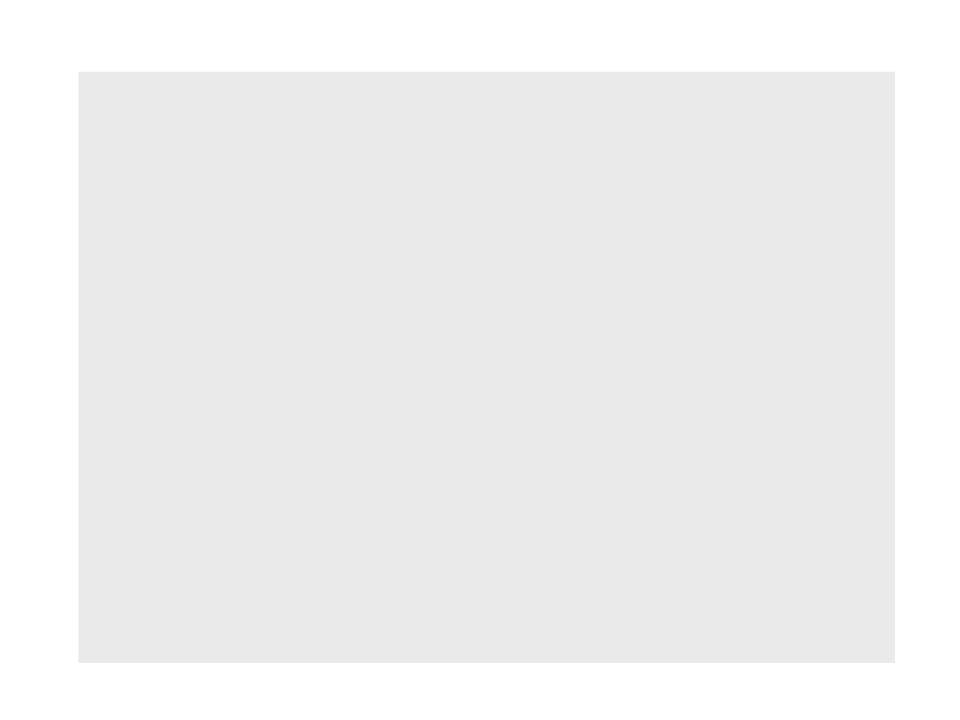local px -- position of the text in the x axis
love.graphics.setColor(0, 0, 0)
love.graphics.setBackgroundColor(1, 1, 1)
px = 0
end
function love.update(dt)
px = px + (100 * dt)
if px > love.graphics.getWidth() then
px = 0
end
end
function love.draw()
love.graphics.print("Hello World", px, 300)
endlocal px -- position of the text in the x axis
love.graphics.setColor(0, 0, 0)
love.graphics.setBackgroundColor(1, 1, 1)
px = 0
end
function love.update(dt)
px = px + (100 * dt)
if px > love.graphics.getWidth() then
px = 0
end
end
function love.draw()
for y = 0, 20, 1 do
love.graphics.print("Hello World", px, y * 30)
end
end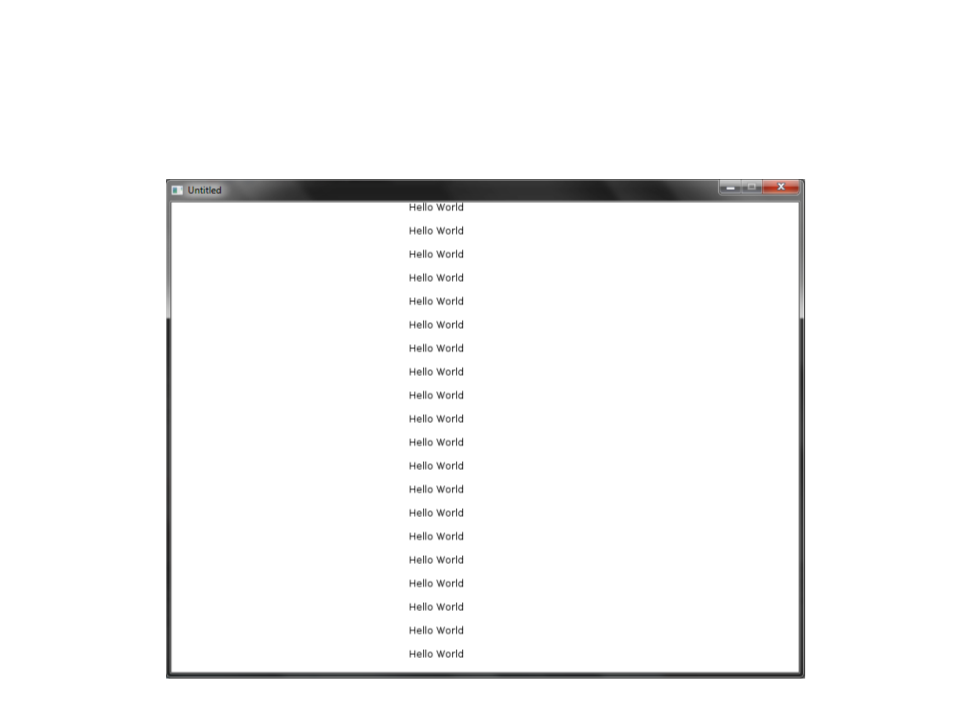Back to the “Hello World”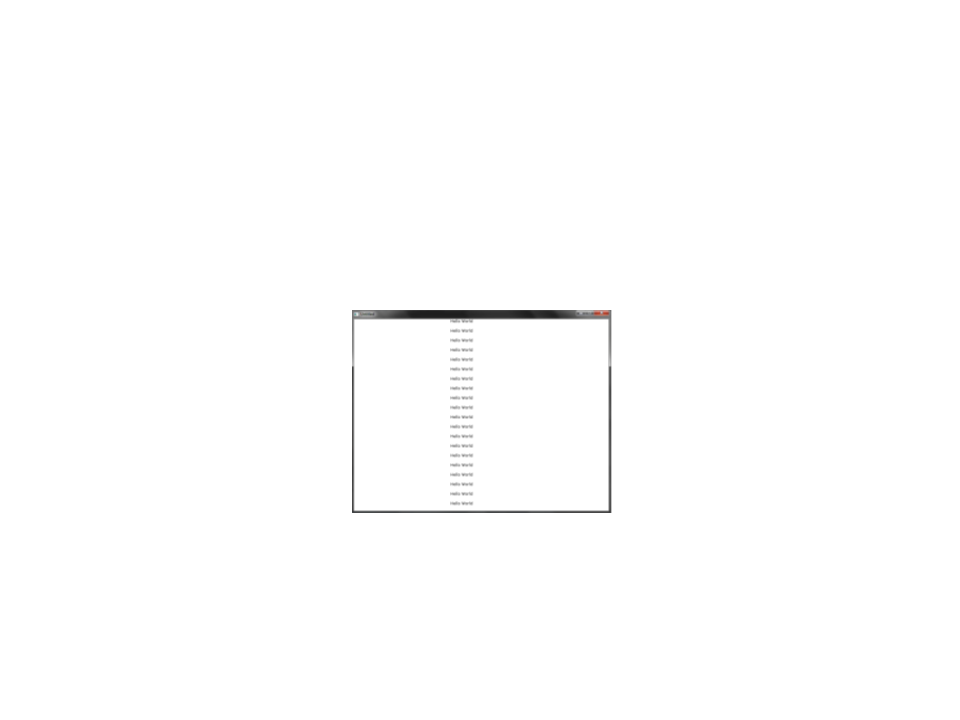Back to the “Hello World”
In the last implementation of the “Hello World”, we
implemented the movement of 20 “Hello World’s”.
Problem: the 20 “Hello World’s” moved all together
How can we make the “Hello World’s” move independently of
each other?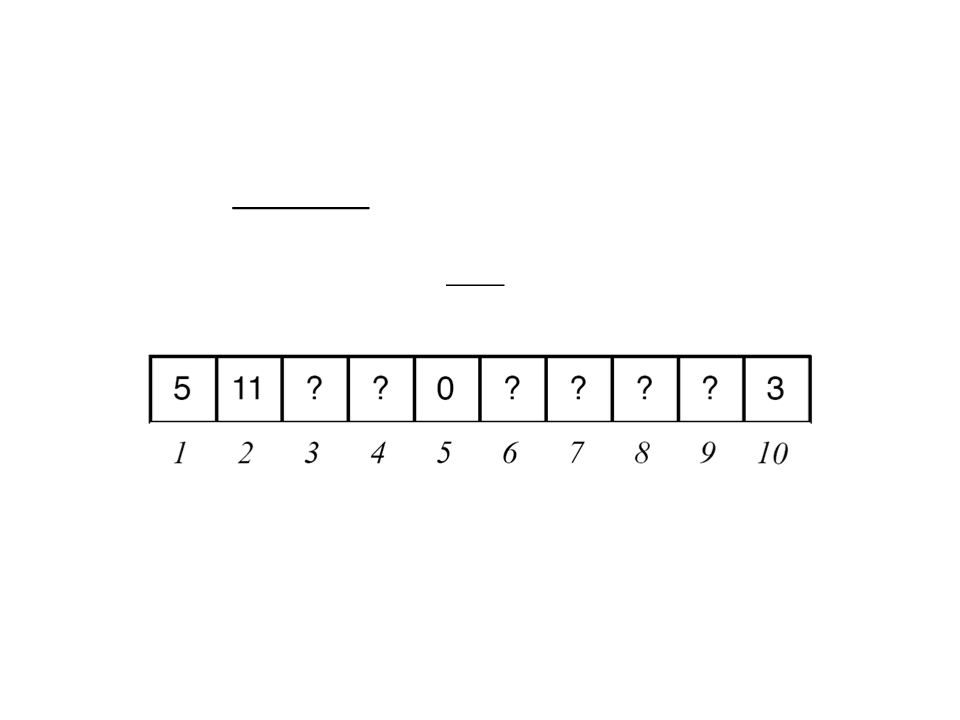Arrays
Arrays are sequences of items (like variables) of the same
type.
Each item is identified by an index (integer).
With arrays we can store in memory sequences of values
numbers, text, imagens, etc.), which are all associated with a
single variable (the array).
(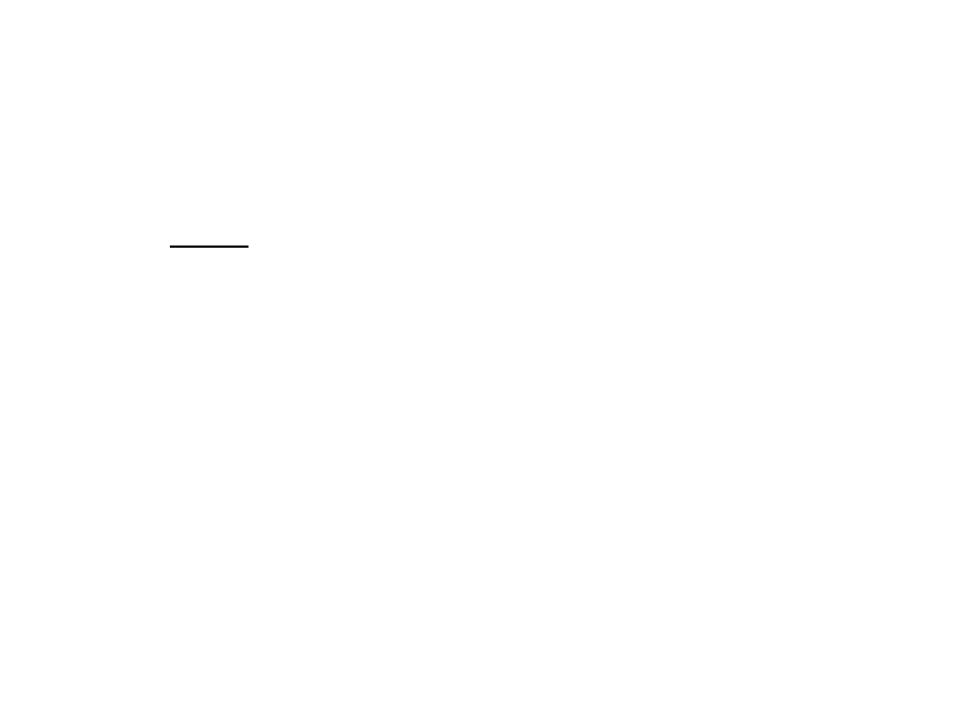Lua Tables
In Lua, all data structures, including arrays, are implemented
using tables.
Tables are the main (in fact, the only) data structuring
mechanism in Lua (a very powerful one).
A Lua table is defined by a set of pairs key-data, where the
data is referenced by the key.
The key (index) can be of any type of data (except nil).Arrays in Lua
In Lua, are implemented through tables indexed by integer
numbers.
Different from many other programming languages, in Lua we
don't need to define the maximum size of an array.
Creating a new array:
my_array = {}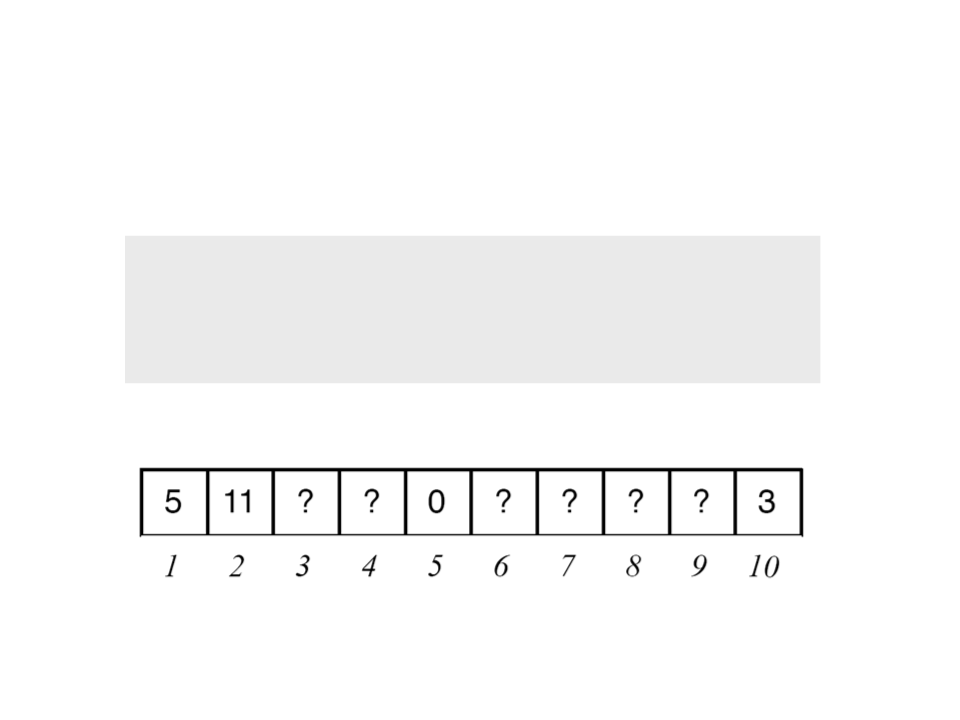Arrays in Lua
Initializing some positions of the array:
my_array = 5
my_array = 11
my_array = 0
my_array = 3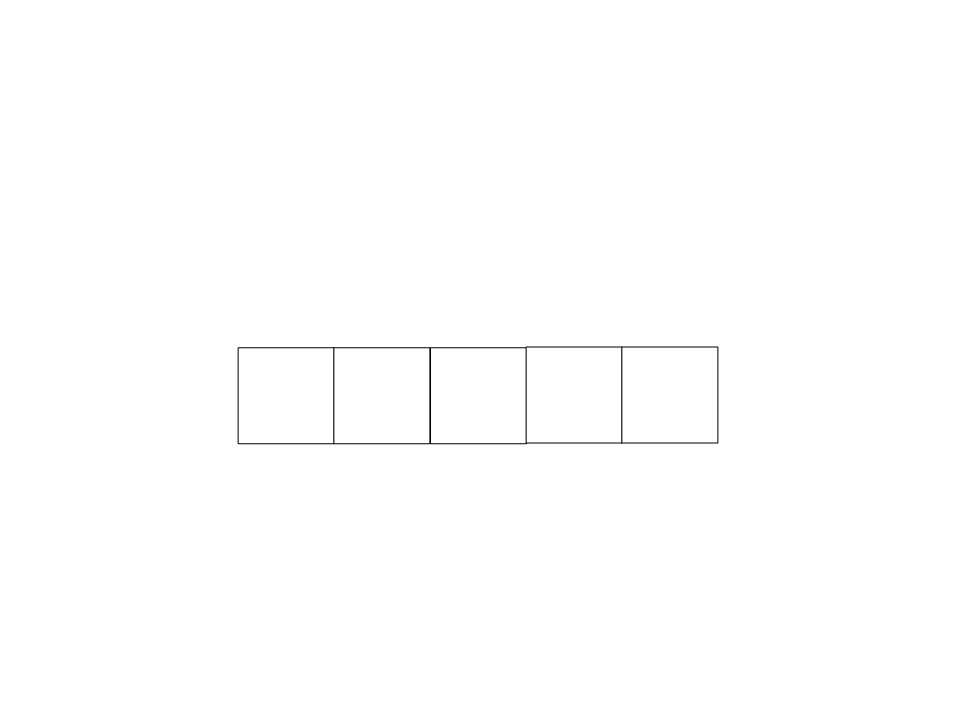Arrays in Lua
We can access the values stored in the array using their index.
myarray = {}
1
2
3
4
5
5
?
?
?
8?
?1
myarray = 5
myarray = 8
myarray = 1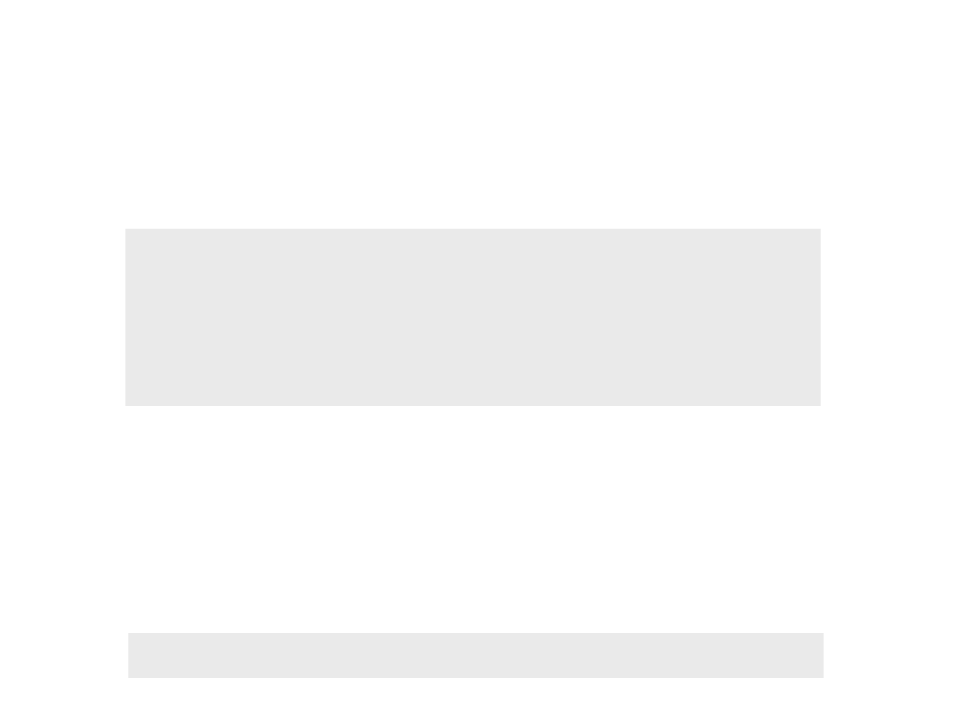Arrays in Lua
Declaring and initializing an array:
a = {} -- new array
for i=1, 1000, 1 do
a[i] = 0
end
Indirectly we are defining that the maximum size of the array
is 1000.
We can also declare and initialize an array using a single
statement:
squares = {1, 4, 9, 16, 25, 36, 49, 64}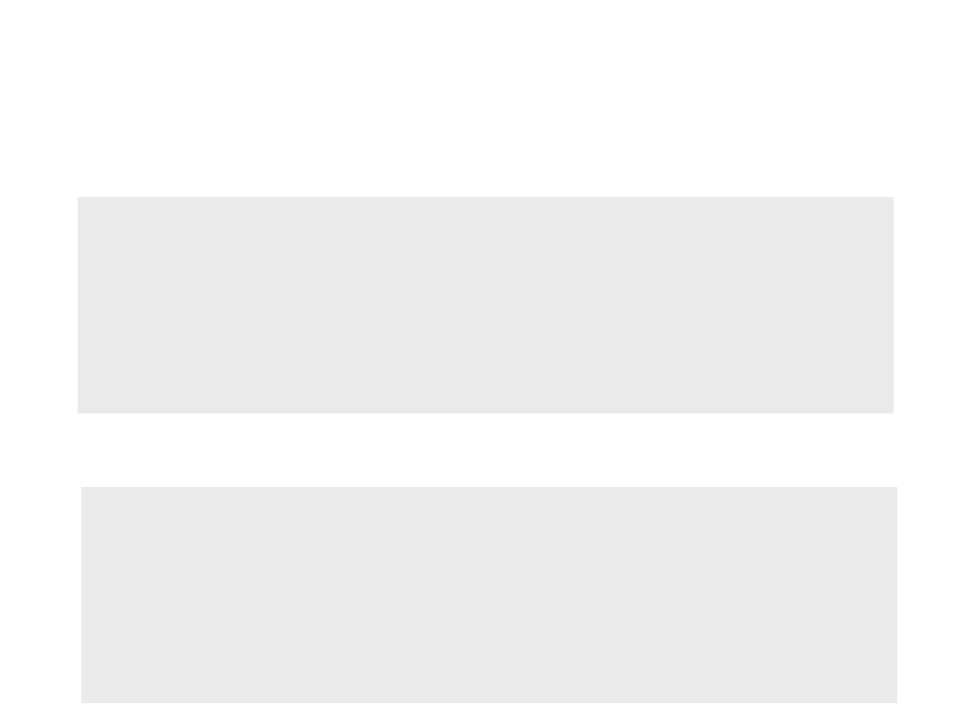Example 1: Displaying the Values of an Array
myarray = {2.3, 5.4, 1.0, 7.6, 8.8, 3.9}
for x = 1, 6, 1 do
io.write(myarray[x], "\n")
end
Inverse order?
myarray = {2.3, 5.4, 1.0, 7.6, 8.8, 3.9}
for x = 6, 1, -1 do
io.write(myarray[x], "\n")
end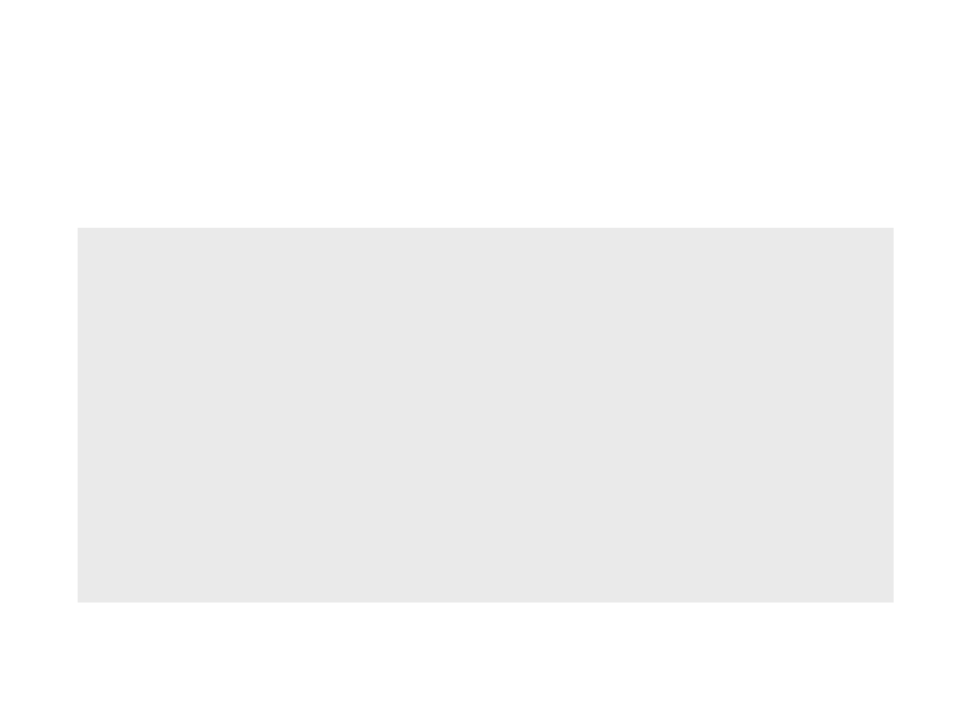Example 2: Sum of the Values of an Array
myarray = {2.3, 5.4, 1.0, 7.6, 8.8, 3.9}
total = 0
for x = 1, 6, 1 do
total = total + myarray[x]
end
io.write(total)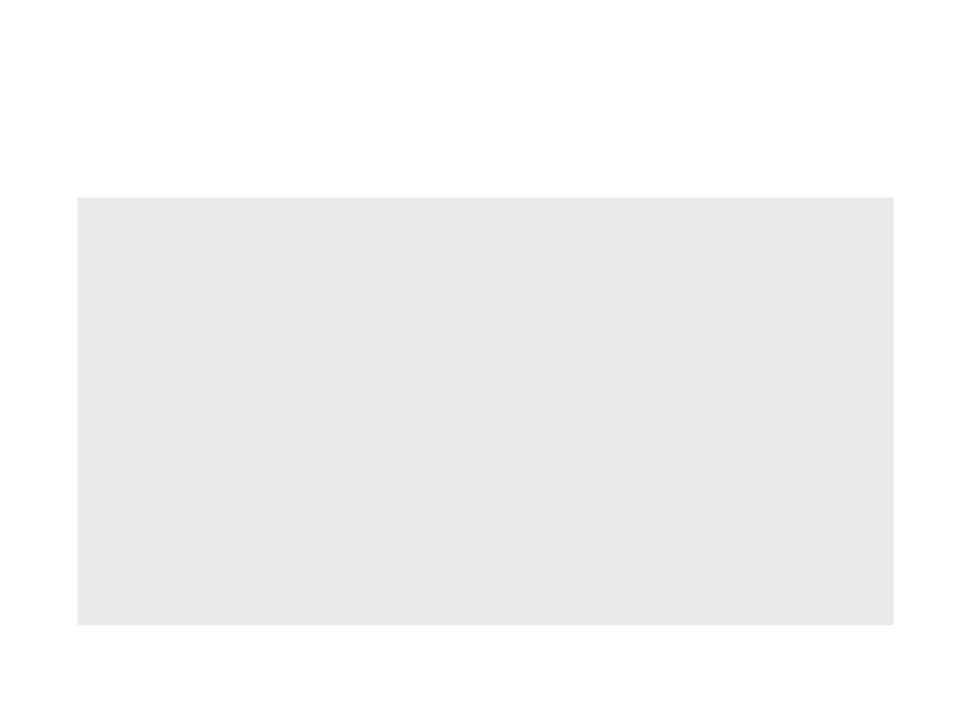Exemplo 3: Finding the Larger Value
myarray = {2.3, 5.4, 1.0, 7.6, 8.8, 3.9}
largervalue = myarray
for x = 2, 6, 1 do
if myarray[x] > largervalue then
largervalue = myarray[x]
end
end
io.write(largervalue)Back to the “Hello World”
In the last implementation of the “Hello World”, we
implemented the movement of 20 “Hello World’s”.
Problem: the 20 “Hello World’s” moved all together
How can we make the “Hello World’s” move independently of
each other?local px
-- position of the text in the x axis
love.graphics.setColor(0, 0, 0)
love.graphics.setBackgroundColor(1, 1, 1)
px = 0
end
function love.update(dt)
px = px + (100 * dt)
if px > love.graphics.getWidth() then
px = 0
end
end
function love.draw()
for y = 0, 20, 1 do
love.graphics.print("Hello World", px, y * 30)
end
end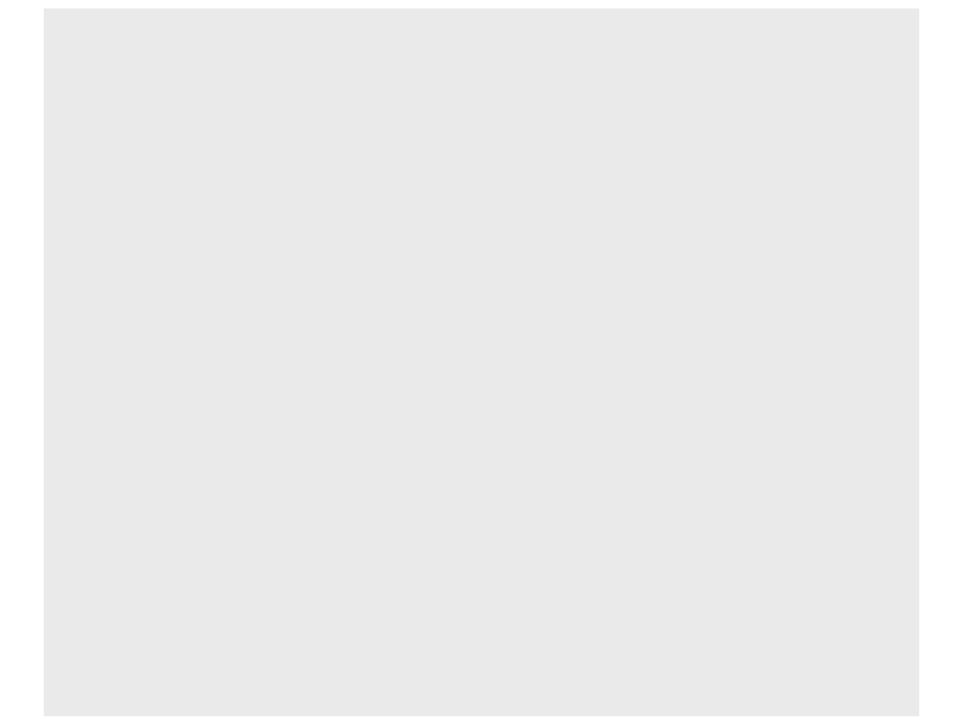local array_px = {} -- array of positions in the x axis
love.graphics.setColor(0, 0, 0)
love.graphics.setBackgroundColor(1, 1, 1)
for y = 1, 20, 1 do
array_px[y] = love.math.random(0, 800)
end
end
function love.update(dt)
for y = 1, 20, 1 do
array_px[y] = array_px[y] + (100 * dt)
if array_px[y] > love.graphics.getWidth() then
array_px[y] = 0
end
end
end
function love.draw()
for y = 1, 20, 1 do
love.graphics.print("Hello World", array_px[y], y * 30)
end
end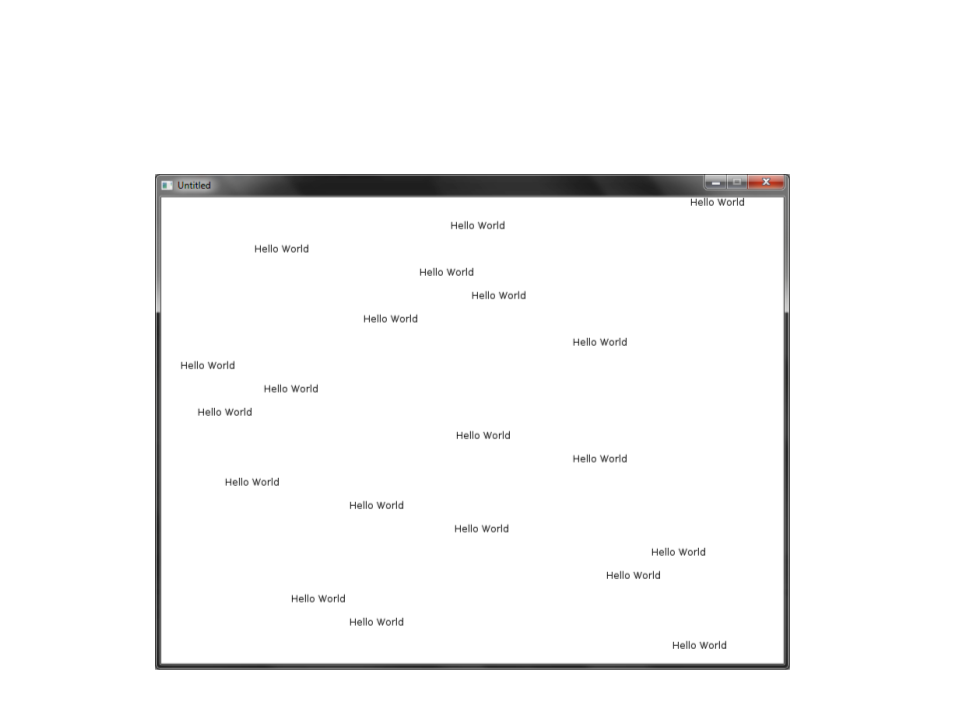Back to the “Hello World”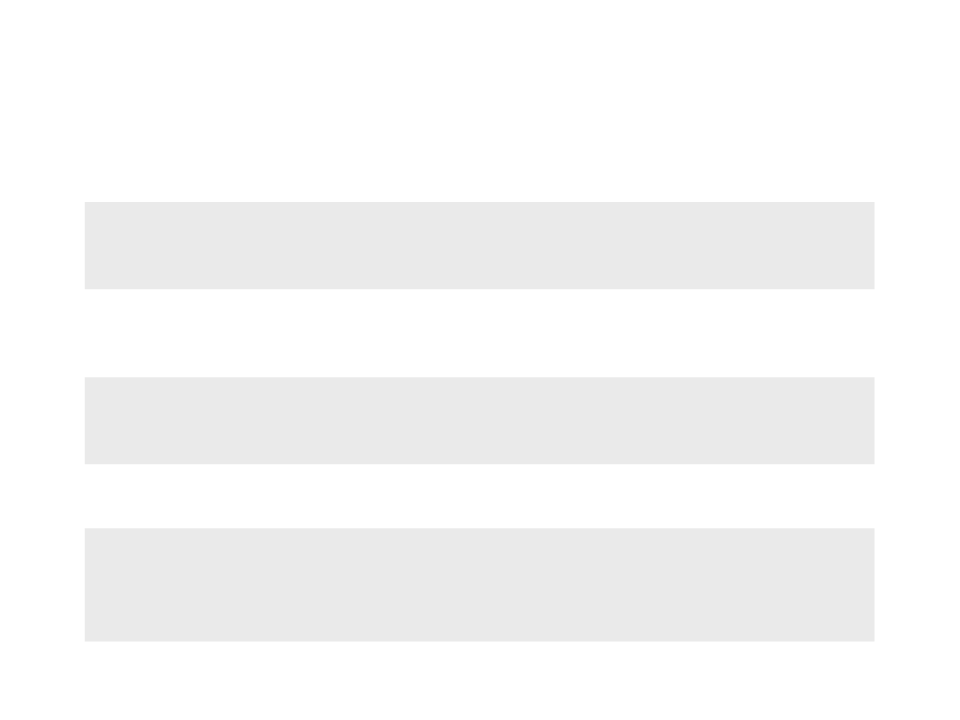Generating Random Numbers
number = love.math.random(min, max)
Example 1:
x = love.math.random(1, 100) -- generates a number between 1
and 100
Exemplo 2:
vet = {} -- fill an array with 100 random numbers
for i=1, 100, 1 do
vet[i] = love.math.random(1, 100)
end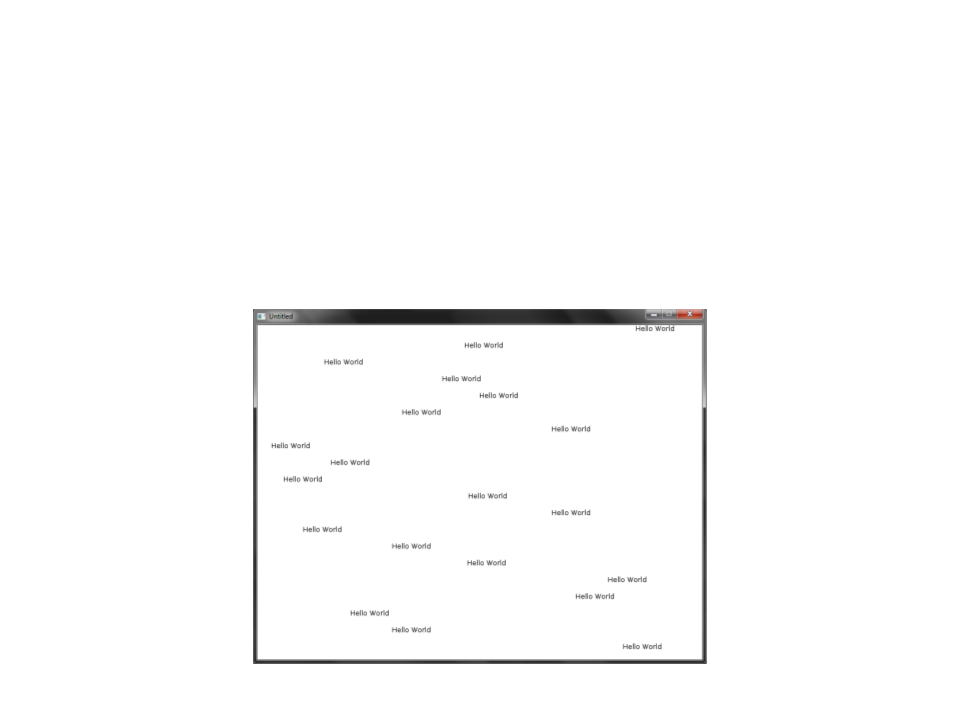Exercise 1
1
) Modify the code of the “Hello World” in order to have each
“Hello World” moving with a different speed (randomly
chosen).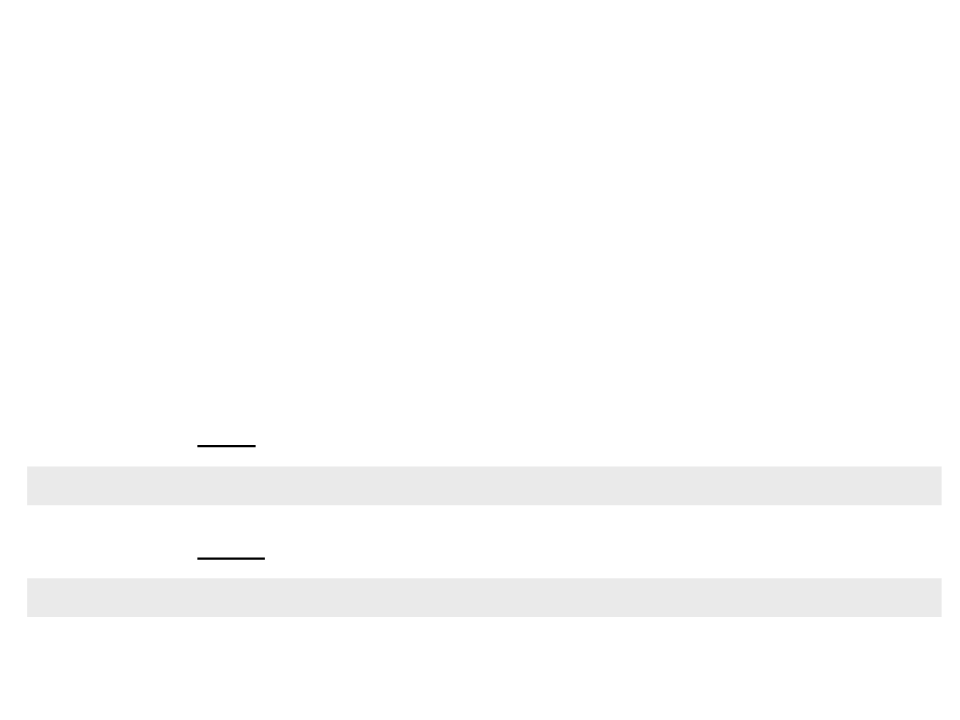ImageType
Games are not created using only geometric shapes. Usually
2
D games use images to represent characters, objects and
environments.
Löve offers a especial data type called image.
We can load a new image using the function:
image = love.graphics.newImage(filename)
We can draw an image using the function:
love.graphics.draw(drawable, x, y, r, sx, sy, ox, oy, kx, ky)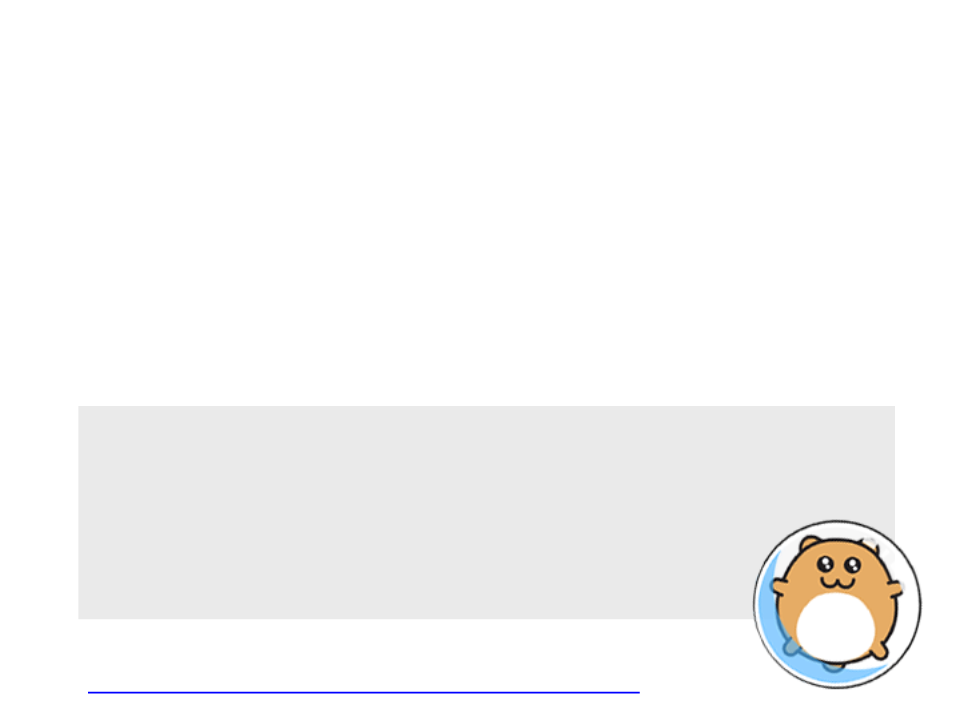ImageType
To draw an image on screen, two steps are required:
Load the image with the function love.graphics.newImage
Draw the image with the function love.graphics.draw
Example:
hamster = love.graphics.newImage("hamster.png")
end
function love.draw()
love.graphics.draw(hamster, 325, 225)
end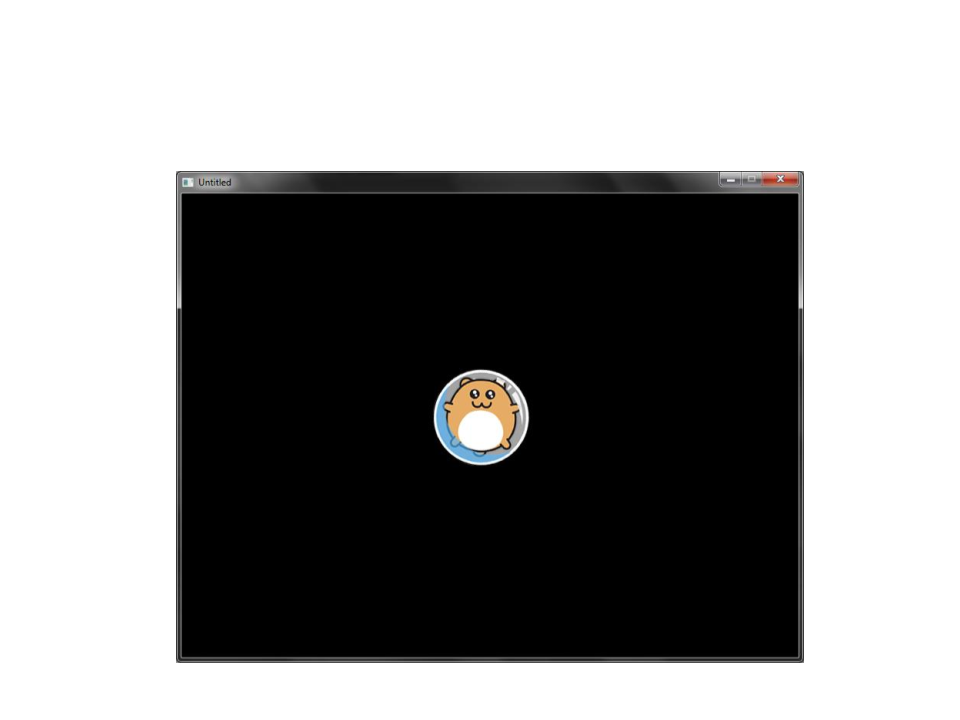ImageType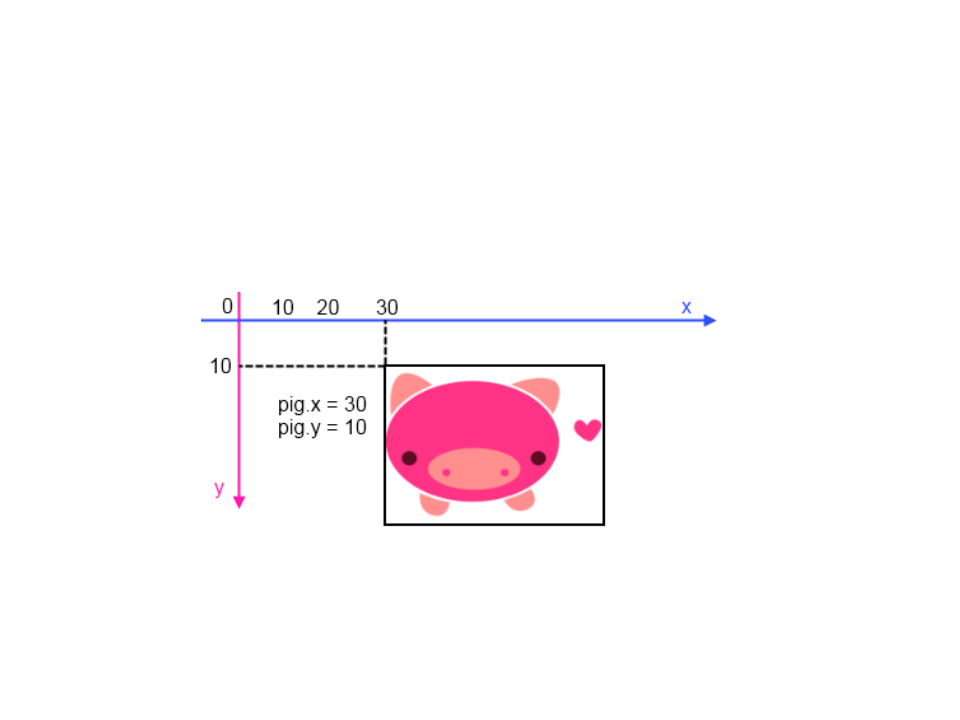ImageType
By default, images are drawn with the origin located at the
top left corner:
Is possible to change the origin point with some addicional
parameters of the love.graphics.drawfunction.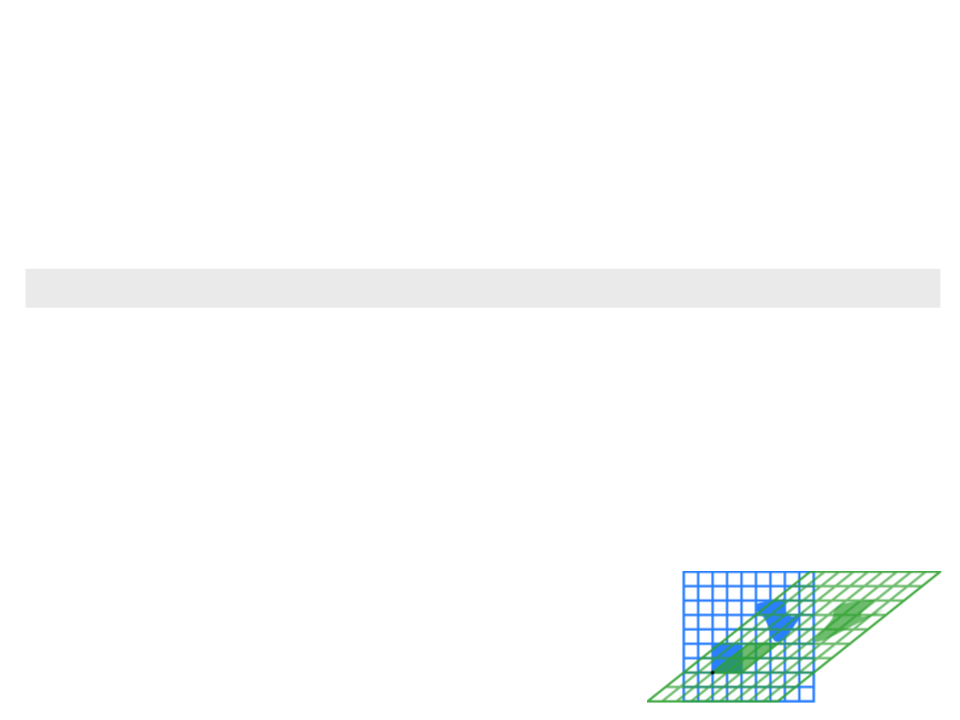ImageType
The function love.graphics.drawhas several optional
parameters:
love.graphics.draw(drawable, x, y, r, sx, sy, ox, oy, kx, ky)
drawable: image or other object that can be drawn on screen;
x: position to draw the object (x-axis);
y: position to draw the object (y-axis);
r: orientation of the object (radians).
sx: scale factor (x-axis);
sy: scale factor (y-axis);
What is shearing fator?
ox: origin offset (x-axis);
oy: origin offset (y-axis);
kx: shearing factor (x-axis).
ky: shearing factor (y-axis).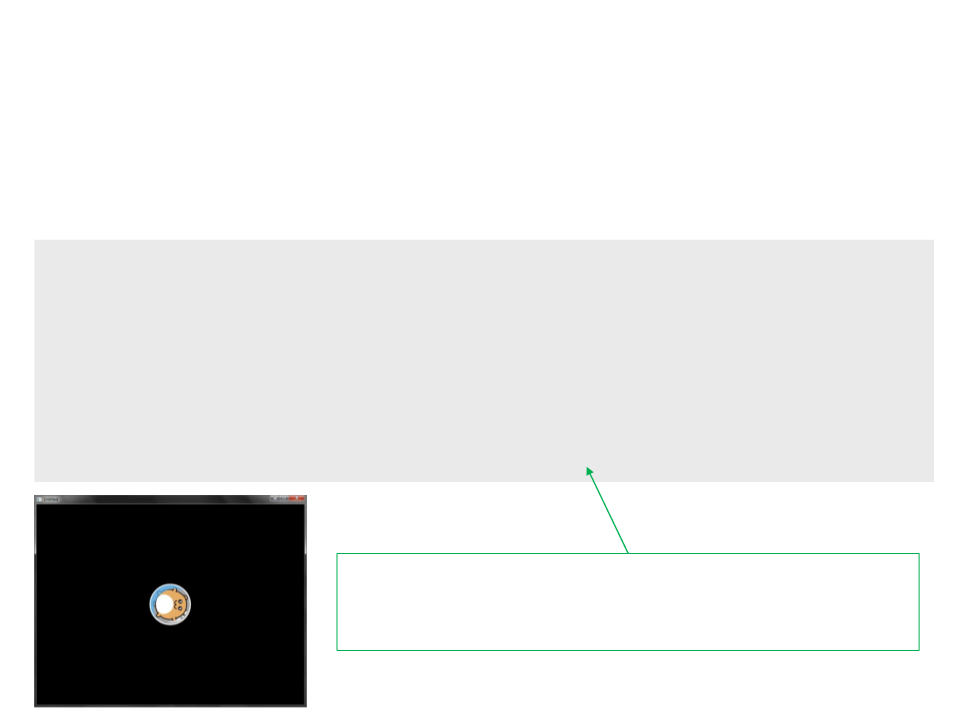ImageType
Example:
hamster = love.graphics.newImage("hamster.png")
end
function love.draw()
love.graphics.draw(hamster, 400, 300, math.rad(90), 1, 1,
hamster:getWidth()/2, hamster:getHeight()/2)
end
Notice that we can get the width and height of an image
with the functions hamster:getWidth()and
hamster:getHeight()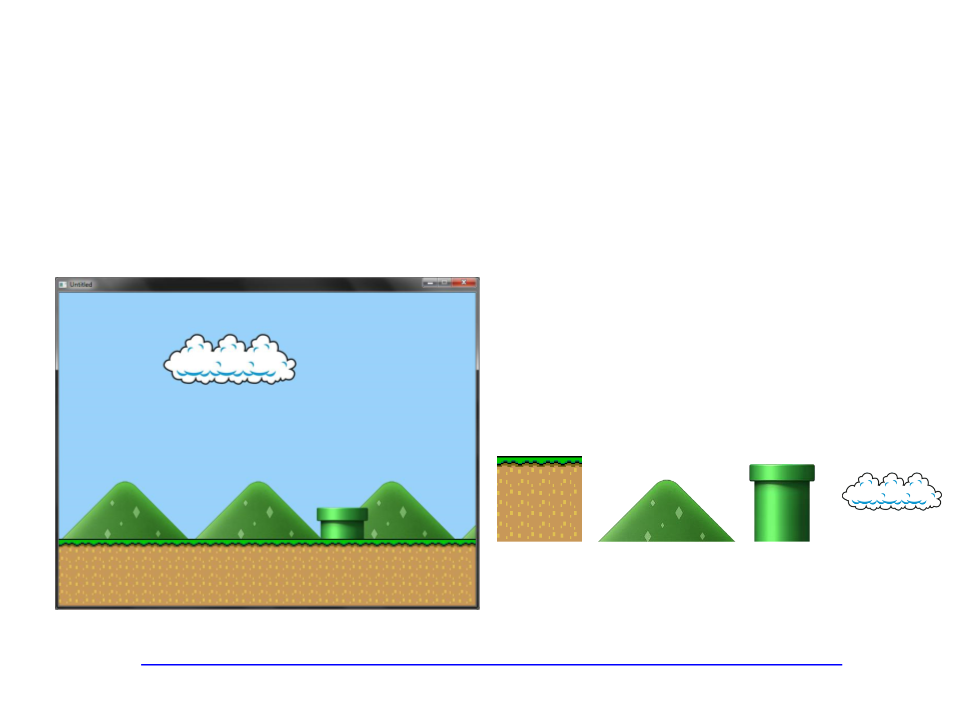Exercise 2
2
) Write a program to draw a scene like the one illustrated
bellow:
Important: you need to use loop statements
to drawn the images that are repeated
several time (ground and mountains).